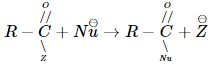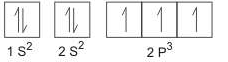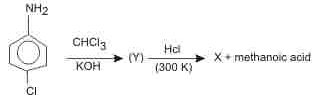Courses

# AFMC Chemistry Mock Test - 2

## 50 Questions MCQ Test AFMC Mock Test Series | AFMC Chemistry Mock Test - 2

Description
This mock test of AFMC Chemistry Mock Test - 2 for NEET helps you for every NEET entrance exam. This contains 50 Multiple Choice Questions for NEET AFMC Chemistry Mock Test - 2 (mcq) to study with solutions a complete question bank. The solved questions answers in this AFMC Chemistry Mock Test - 2 quiz give you a good mix of easy questions and tough questions. NEET students definitely take this AFMC Chemistry Mock Test - 2 exercise for a better result in the exam. You can find other AFMC Chemistry Mock Test - 2 extra questions, long questions & short questions for NEET on EduRev as well by searching above.
QUESTION: 1

Solution:
QUESTION: 2

Solution:
QUESTION: 3

### The rate of the reactionis fastest when Z is

Solution:Reactivity of the acid derivatives decreases as the basicity of the leaving group increases. The basicity of the leaving group increases as

Cl^– < RCOO^– < RO^– < NH2^–

Secondly least stabilization by resonance due to ineffective overlapping between the 3p orbital of Cl and 2p orbital of carbon.
QUESTION: 4

The maximum number of electrons in a subshell is given by the expression

Solution:
QUESTION: 5

The Bohr's theory was modified to give the concept of elliptical orbits by

Solution:
QUESTION: 6

Which is an essential constituent of a diet?

Solution:
QUESTION: 7

Identify the correct statement regarding enzymes.

Solution:
QUESTION: 8

Vinegar made from cane sugar, now-a-days synthetically contains

Solution:
QUESTION: 9

In the resonating structure of benzene the number of σ and π bond are

Solution:
QUESTION: 10

A characteristic feature of reversible reactions is that they

Solution:
QUESTION: 11

A cylinder filled with a movable piston contains liquid water in equilibrium with water vapour at 25°C. Which one of the following operations results in a decreace in the equilibrium vapour pressure ?

Solution:
QUESTION: 12

A reaction with respect to the reactant A has a rate constant of 5 s⁻1. If we start with[A]= 10 mol-L⁻1, then in what time the concentration of A becomes 1.0 mol-L⁻1?

Solution:
QUESTION: 13

A catalyst is used to

Solution:
QUESTION: 14

Which of the following is not isoelectronic?

Solution:

Isoelectronic species: a group of ions, atoms, or molecules that have the same number of electrons.
Number of electrons in Na+ = 10
Number of electrons in Mg2+ = 10
Number of electrons in O2 − = 10
Number of electrons in Cl = 18

QUESTION: 15

Which of the following has highest first ionization potential?

Solution:

Nitrogen has highest first ionization potential. This is because N contains exactly half-filled p-orbitals which is extra stable. hence its first ionisation potential is high.
Electronic configuration of N:QUESTION: 16

Which of the following compound contains both the oxidiser and the fuel elements in the same molecule?

Solution:
QUESTION: 17

An adiabatic expansion of an ideal gas always has

Solution:
QUESTION: 18

Azo dyes are prepared from

Solution:
QUESTION: 19

Of the five isomeric hexane,the isomers which can give two monochlorimated compound is

Solution:
QUESTION: 20

The pH of aqueous KCl solution is 7.0. This solution was electrolysed for a few seconds using Pt electrodes. Which of the following is correct?

Solution: Option B is correct becz during electrolysis, at anode there is oxidation of Cl minus ions (among Cl minus and OH minus present in soln. ) liberating Cl2 while on cathode there is reduction of H plus ions (among k plus & H plus in soln.) liberating H2, leaving OH minus and k+ ions in the soln this OH minus ion is responsible for increasing pH of soln.
QUESTION: 21

The reaction 1/2 H₂(g) + AgCl(s) → H⁺(aq) + Cl- (aq) + Ag(s) occurs in the galvanic cell

Solution:
QUESTION: 22

Cassiterite is an ore of

Solution:
QUESTION: 23

Which of the following has the highest conductivity?

Solution:
QUESTION: 24

Which halide has highest melting point?

Solution: More the ionic character,more the melting point.So NaF is having highest ionic character because ,the electronegativity of fluorine is the highest hence it have the greatest pull or attraction towards the electrons in the ionic bond , resulting in a really strong sodium positive ion and a strong negative fluorine ion.
QUESTION: 25

A metal X on heating in nitrogen gas gives Y. Y on treatment with H₂O gives a colourless gas which when passed through CuSO₄ solution gives a blue colour. Y is

Solution:
QUESTION: 26

Which of the following compounds is acidic

Solution:
QUESTION: 27

1-Chlorobutane on reaction with alcoholic potash gives

Solution:
QUESTION: 28

Hardness of water is due to which pair of ions?

Solution:
QUESTION: 29

Which of the following is a weak electrolyte?

Solution: NH4OH doesn't dissociates completely leading to its weak basic character. ... [NaOH=Na+ + OH- ].On the other handNH4OH partially disaccociates in water for which concentration of (OH-)ion in aqueous solution is less,making it a weakbase.[NH4OH=NH4+ + OH-].
QUESTION: 30

Isotopes of elements were discovered by

Solution:
QUESTION: 31

In case of a radioisotope, the value to T1/2 and λ are identical in magnitude. The value is

Solution:
QUESTION: 32

Heaviest particle is

Solution:
QUESTION: 33

Which of the following is called Sandmeyer reaction ?

Solution:
QUESTION: 34

When ammonia is passed over heated copper oxide, the metallic copper is obtained. The reaction show that ammonia is

Solution:
QUESTION: 35

Which of the following statement is correct with respect to the property of elements with increase in atomic no. in the carbon family?

Solution:
QUESTION: 36

Which of the following is a nitric anhydride?

Solution:
QUESTION: 37

The strongest base among the following is

Solution:
QUESTION: 38

When potassium ferrocyanide crystals are heated with conc. H₂SO₄, the gas evolved is

Solution:
QUESTION: 39

The process of volcanisation was introduced by

Solution:
QUESTION: 40

Which of the following is not a synthetic polymer?

Solution:
QUESTION: 41

Which of the following radicals can bring about the highest oxidation state of a transition metal ?

Solution:
QUESTION: 42

1.0 g of pure calcium carbonate was found to require 50 ml of dilute HCl for complete reaction. The strength of HCl solution is given by

Solution:
QUESTION: 43

The osmotic pressure of 5% (mass-volume) solution of cane sugar at 150°C (mol. mass of sugar = 342) is

Solution:
QUESTION: 44

IdentifyX in the sequence given below :Solution:
QUESTION: 45

The density of a gas at 27°C and 1 atm is d. Pressure remaining constant, at which of the following temperatures will its density become 0.75d?

Solution:
QUESTION: 46

An ideal gas cannot be liquified because

Solution:
QUESTION: 47

The reactions in zeolite catalysts depend upon

Solution:
QUESTION: 48

Transition metals are often paramagnetic owing to

Solution:
QUESTION: 49

Titanium shows magnetic moment of 1.73 B.M. in its compound. What is the oxidation number of Ti in the compound ?

Solution:
QUESTION: 50

Rusting of iron is catalysed by

Solution:

Track your progress, build streaks, highlight & save important lessons and more!

### Similar Content### Related tests